# Discussion Session 2¶

## Grammar¶

• Nonterminal
• Terminal
• Production Rule (Left Hand Side, Right Hand Side)

Example

start ::=  exp            // Start
exp   ::=  exp + term   // Add
exp   ::=  exp - term   // Minus
exp   ::=  term           // Term
term  ::=  ID              // Id
term  ::=  NUM             // Num
term  ::=  (exp)         // Group


## Parsing¶

Parsing is the procedure of transforming a list of tokens into a tree with tokens as leaves.

Note

This process is also called derivation.Token stream t1, t2, t3, t4, t5, t6, ...... and Parsing Tree (Abstract Syntax)

Color Meaning
Red Terminal
Blue Nonterminal

Example

The abstract syntax for 1 - 2 - 3 -4 goes as follows: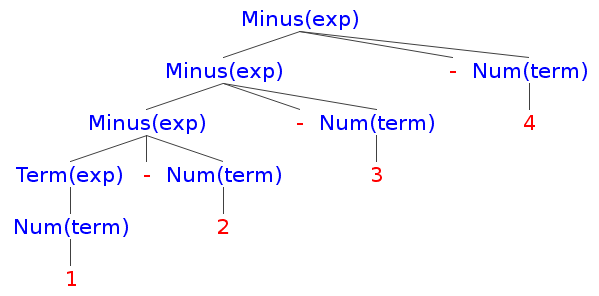• A good grammar has no ambiguity. Only one tree can be constructed from the token stream.

• A good grammar should match human expectation.

Example

Try the following grammar on 1 - 2 - 3 - 4.

start ::=  exp           // Start
exp   ::=  term + exp    // Add
exp   ::=  term - exp    // Minus
exp   ::=  term            // Term
term  ::=  ID               // Id
term  ::=  NUM              // Num
term  ::=  (exp)          // Group


## Anatomy of LL(k)¶

L: Left-to-right

Examine the input (token stream) left-to-right in one parse.

L: Leftmost-derivation

Create / expand the left most sub-tree first.

Note

R: Rightmost-derivation

demo

Try parsing “1 + 2 - 3 * 4 + 5” with the following grammar.

start ::=  exp            // Start
exp   ::=  exp + term   // Add
exp   ::=  exp - term   // Minus
exp   ::=  term           // Term
term  ::=  term * NUM    // Mul
term  ::=  term / NUM    // Div
term  ::=  NUM             // Num
term  ::=  (exp)         // Group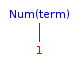Step 1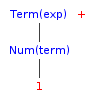Step 2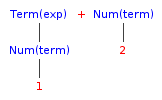Step 3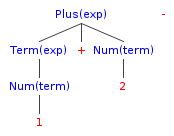Step 4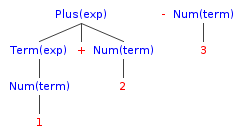Step 5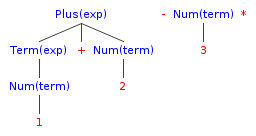Step 6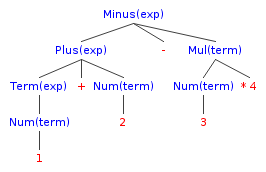Step 7

Inspect the first k tokens before making decision.

## Eliminating Left Recursion¶

For productions like this:

$P \rightarrow P\alpha_1 \mid P\alpha_2 \mid \cdots \mid P\alpha_m \mid \beta_1 \mid \cdots \mid \beta_n$$\textrm{where } \alpha \neq \varepsilon, \beta \textrm{ don't start with } P$

It will be turned into

$P \rightarrow \beta_1 P' \mid \beta_2 P' \mid \cdots \mid \beta_n P'$$P' \rightarrow \alpha_1 P' \mid \alpha_2 P' \mid \cdots \mid \alpha_m P' \mid \varepsilon$

And you can verify that the resulting language is the same.

Warning

This is actually eliminating direct left recursions, and turning them into right recursions. There are methods to eliminate all recursions, direct or indirect, but it is more complicated, and needs some restrictions on the input grammar.

Example

start ::=  exp            // Start
exp   ::=  exp + term   // Add
exp   ::=  exp - term   // Minus
exp   ::=  term           // Term
term  ::=  ID              // Id
term  ::=  NUM             // Num
term  ::=  (exp)         // Group


is turned into

start ::=  exp
exp   ::=  term exp1
exp1  ::=  + term exp1
exp1  ::=  - term exp1
exp1  ::=  epsilon


## Left-factoring¶

For productions like this:

$A \rightarrow \delta\beta_1 \mid \delta\beta_2 \mid\cdots\mid\delta\beta_n \mid \gamma_1 \mid \cdots \mid \gamma_m$

We turn them into

$A \rightarrow \delta A' \mid \gamma_1 \mid \cdots \mid \gamma_m$$A' \rightarrow \beta_1 \mid \cdots \mid \beta_n$

Example

start ::=  exp          // Start
exp   ::=  exp + term   // Add
exp   ::=  exp - term   // Minus
exp   ::=  term           // Term
term  ::=  ID              // Id
term  ::=  NUM             // Num
term  ::=  (exp)         // Group


is turned into

start ::=  exp
exp   ::=  exp term1
term1 ::=  + term
term1 ::=  - term
exp   ::=  term


Do left recursion elimination.

start ::=  exp
exp   ::=  term exp1
exp1  ::=  term1 exp1
exp1  ::=  epsilon
term1 ::=  + term
term1 ::=  - term